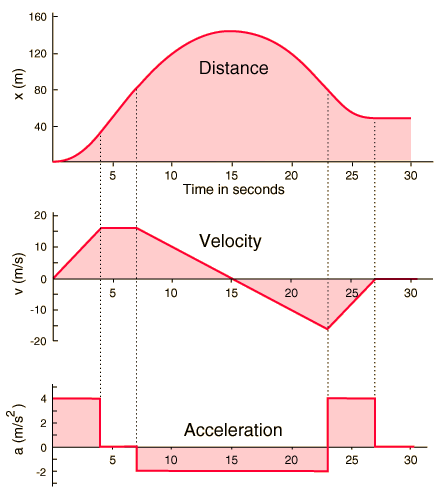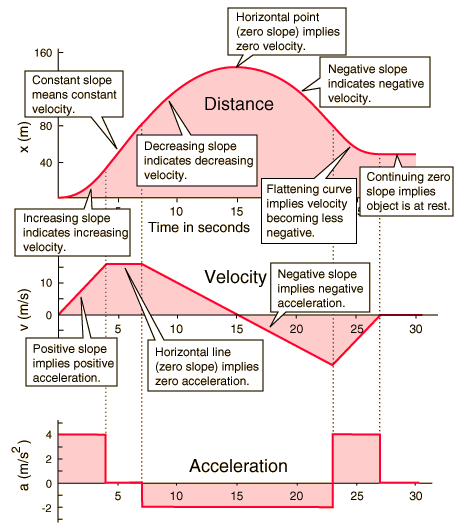Graphing Motion

The graphs of distance, velocity and acceleration as functions of time below were calculated for one-dimensional motion using the motion equations in a spreadsheet. The acceleration does change, but it is constant within a given time segment so that the constant acceleration equations can be used. For variable acceleration (i.e., continuously changing), then calculus methods must be used to calculate the motion graphs.